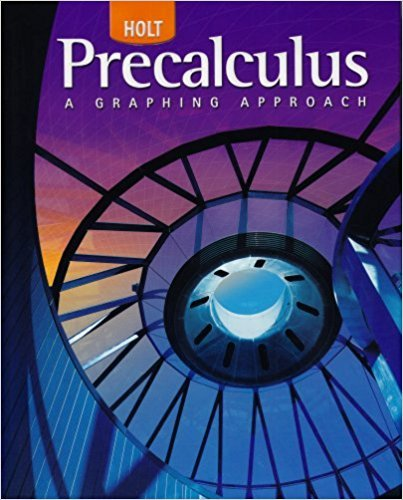×
×

# Solutions for Chapter 3.1: Functions## Full solutions for Precalculus | 1st Edition

ISBN: 9780030416477Solutions for Chapter 3.1: Functions

Solutions for Chapter 3.1
4 5 0 247 Reviews
18
2
##### ISBN: 9780030416477

Precalculus was written by and is associated to the ISBN: 9780030416477. This expansive textbook survival guide covers the following chapters and their solutions. Chapter 3.1: Functions includes 72 full step-by-step solutions. This textbook survival guide was created for the textbook: Precalculus, edition: 1. Since 72 problems in chapter 3.1: Functions have been answered, more than 26247 students have viewed full step-by-step solutions from this chapter.

Key Calculus Terms and definitions covered in this textbook
• Arccotangent function

See Inverse cotangent function.

• Determinant

A number that is associated with a square matrix

• Direct variation

See Power function.

• Directed line segment

See Arrow.

• Elimination method

A method of solving a system of linear equations

• Equally likely outcomes

Outcomes of an experiment that have the same probability of occurring.

• Linear inequality in x

An inequality that can be written in the form ax + b < 0 ,ax + b … 0 , ax + b > 0, or ax + b Ú 0, where a and b are real numbers and a Z 0

• Linear programming problem

A method of solving certain problems involving maximizing or minimizing a function of two variables (called an objective function) subject to restrictions (called constraints)

• Maximum r-value

The value of |r| at the point on the graph of a polar equation that has the maximum distance from the pole

• nth root

See Principal nth root

• Ordered set

A set is ordered if it is possible to compare any two elements and say that one element is “less than” or “greater than” the other.

• Parabola

The graph of a quadratic function, or the set of points in a plane that are equidistant from a fixed point (the focus) and a fixed line (the directrix).

• Partial fraction decomposition

See Partial fractions.

• Rational numbers

Numbers that can be written as a/b, where a and b are integers, and b ? 0.

• Rational zeros theorem

A procedure for finding the possible rational zeros of a polynomial.

• Reduced row echelon form

A matrix in row echelon form with every column that has a leading 1 having 0’s in all other positions.

• Regression model

An equation found by regression and which can be used to predict unknown values.

• Right angle

A 90° angle.

• Standard representation of a vector

A representative arrow with its initial point at the origin

• Zero of a function

A value in the domain of a function that makes the function value zero.

×